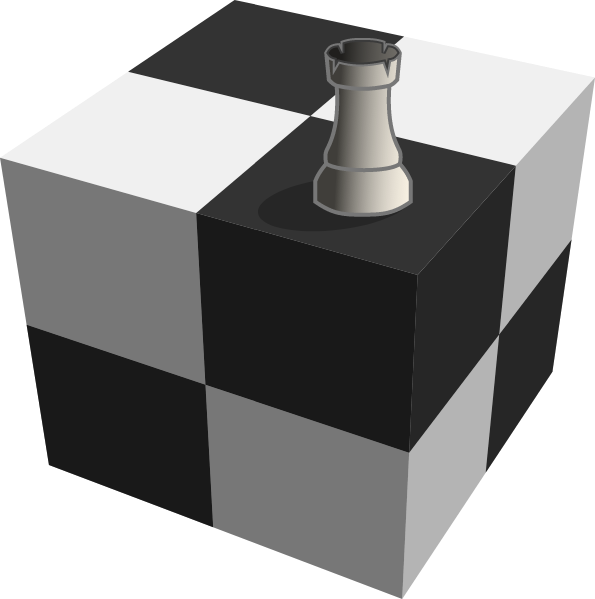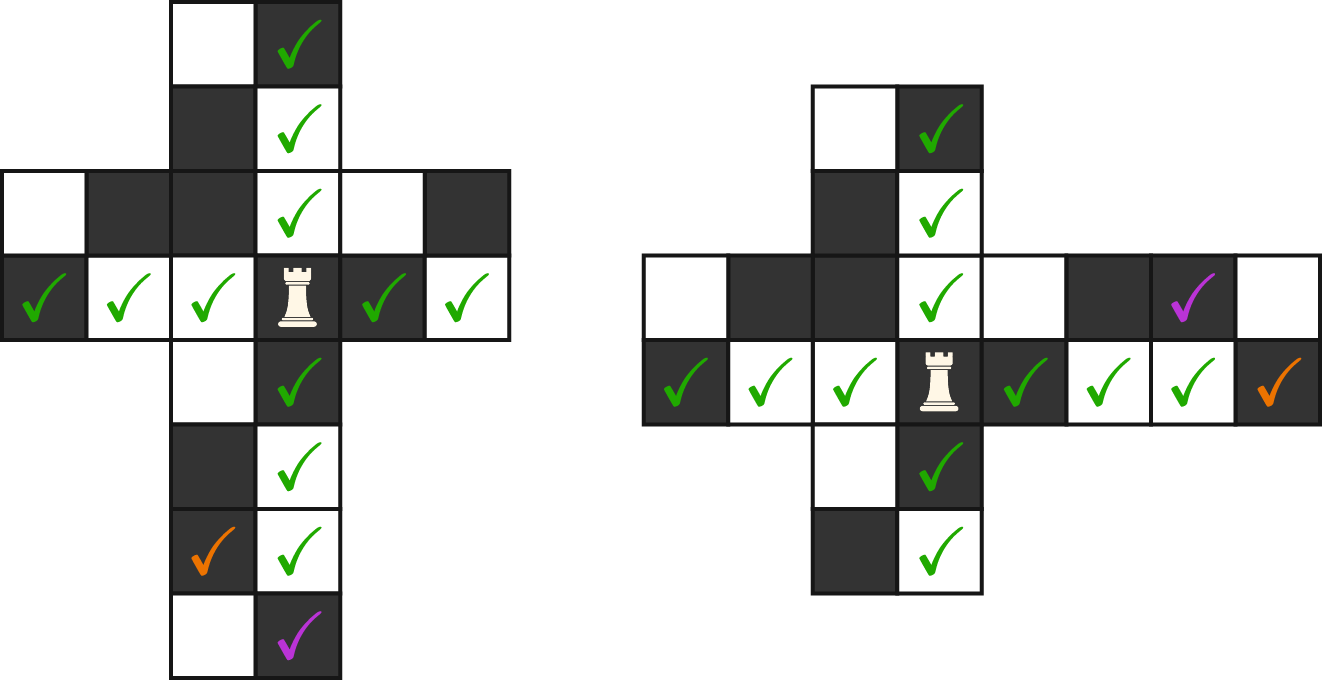# Queen on a cube

Probability Level 2Suppose we're playing chess on a $2 \times 2 \times 2$ cube, where the movements of pieces that are considered valid are those that would be valid on a flattened-out net. For example, if a rook is placed on a square, it could reach 13 other squares in one move.

If a queen is placed on a square, how many squares could it reach in one move?The space marked orange is not accessible on the left net, but it is accessible on the right net. Likewise, the space marked purple is accessible on the left net, but is not accessible on the right net. There are 13 accessible spaces in all.

Note: On a $2 \times 2 \times 2$ cube, each of the squares can equivalently be the starting position. As shown above, as long as a space is accessible on at least one net, it is considered a valid movement.

×